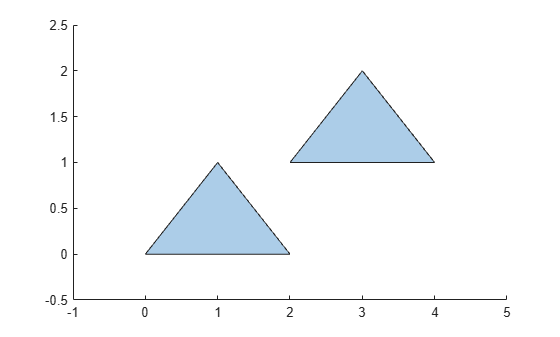# numboundaries

Number of `polyshape` boundaries

## Syntax

``N = numboundaries(polyin)``

## Description

example

````N = numboundaries(polyin)` returns the number of boundaries of a `polyshape` object.```

## Examples

collapse all

Create a polygon and determine its number of boundaries.

```x1 = [0 1 2]; y1 = [0 1 0]; x2 = [2 3 4]; y2 = [1 2 1]; polyin = polyshape({x1,x2},{y1,y2}); plot(polyin)````N = numboundaries(polyin)`
```N = 2 ```

## Input Arguments

collapse all

Input `polyshape`, specified as a scalar, vector, matrix, or multidimensional array.

Data Types: `polyshape`